# Analyticity of Common Complex Functions

• Difficulty Level : Expert
• Last Updated : 20 Mar, 2022

The analyticity of a complex function is a non-trivial property. However, there exists a large class of functions that are analytic and these functions are our subject of interest in this section.

### Rational Functions

Rational functions are functions that can be expressed as P(z)/Q(z) form, where P(z) and Q(z) are polynomials. Rational functions are analytic everywhere except where Q(z) becomes zero in the complex plane (or the poles of the function). To prove that rational functions are analytic in general, we can try applying Cauchy-Riemann equations on a general rational function with P(z) being a polynomial of degree n, and Q(z) a polynomial of degree m. However, this method is tedious and you might give up in the middle.

An illuminating way to prove this is to observe that analytic functions obey the general laws satisfied by derivatives of real functions, such as the derivative of the sum or the difference of two functions is the sum or difference of the derivatives of the individual functions. Again, we have the familiar product rule. We can write them as:

`[f(z)+g(z)]' = f'(z)+g'(z) and [f(z)g(z)]' = f'(z)g(z)+f(z)g'(z) and [cf(z)]' = cf'(z) .`

Clearly, the powers of z are analytic since z is analytic. And polynomials are sums of powers of z multiplied by constants. Hence they are clearly analytic.

Similarly, we have the quotient rule as well.

`[f(z)/g(z)]' = [f'(z)g(z) - f(z)g'(z)]/g(z)2`

provided that g(z) is not equal to zero. So rational functions are analytic except at the poles of the function.

An interesting thing about analytic functions is that any function containing z* is not analytic. And any rational function is analytic if the z* dependence cancels out from the numerator and denominator. We can check if it’s a valid criterion. Expressing z* = x +iy, we have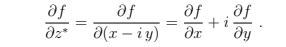Now, let’s separate f into u and v.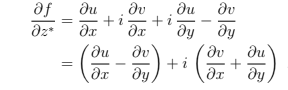So, if f is analytic, then it should be zero. Hence there should not be any z* in f for f to be analytic.

### Complex Exponential

Let z = x + iy be a complex number and define the complex exponential exp(z) or ez as:

`exp(z) = exp(x + i y) = ex(cosy + i siny)`

exp(z) is not only analytic, it is entire. We can decompose exp(z) into real and imaginary parts,

`u(x,y) = excosy and v(x,y) = exsiny`

The Cauchy-Riemann equations are satisfied by exp(z),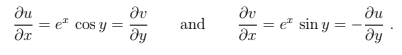So the derivative of exp(z) is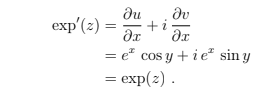Just like the real exponential function!

The exponential function has many properties analogous to the real exponential, such as exp(z1+z2)=exp(z1)exp(z2)

However, there is an interesting feature of the complex exponential with no real analogies- it is periodic with a period of 2πi. This is trivial because, from the definition, we can see that

`exp(z+2πi) = exp(x + i y + 2πi ) = ex[cos(y+2π) + i sin(y+2π)] = ex(cosy + i siny) = exp(z) `

sine and cosine are periodic with periods of 2π.

This implies that the exponential function is not one-to-one. In particular,

`exp(z1) = exp(z2) implies z1 = z2 +2πik, where k ∈ I`

So, we can divide the entire Argand plane into horizontal strips of width 2π, where exp(z) would be one-to-one.

### Complex Trigonometric Functions

We define :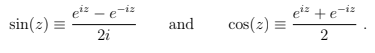They are entire (check!). Plus they share some properties of real sines and cosines.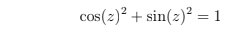However, there is one important difference between the real and complex trigonometric functions: whereas the real sine and cosine functions are bounded between -1 and 1, their complex counterparts are not. To see this let us break them up into real and imaginary parts: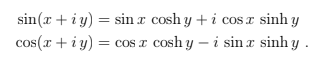We see hyperbolic functions, which are clearly not bound.

### Complex Logarithm

By analogy with the real natural logarithm, we define the complex logarithm as an inverse to the complex exponential function. In other words, we say that a logarithm of a nonzero complex number z is any complex number w such that exp(w) = z. In other words, we define the function log(z) by

`w = log(z) if and only if z = exp(w) .`

From the periodicity of the exponential function, it follows that if w = log(z) so is w + 2πik for any integer k. Therefore we see that log(z) is a multiple-valued function.

In fact, log(z) is multiple-valued due to the multiple-valuedness of the argument function of z arg(z). We know that every complex number can be represented as z = |z|e, where |z| is the magnitude of z and θ=arg(z). Usually, we take the principal value of arg(z), that is, -π<θ≤π. But, any θ+2π would have been as good. In particular, arg(z) = Arg(z) + 2πk , where Arg(z) represents the principal value of arg(z) and k ∈ I

`Now, log(z) = Log|z| + i arg(z) = Log|z| + i Arg(z) + 2πik.`

where Log|z| represents the real logarithmic function because |z| is (obviously) real.

The above definition holds because:

```exp[ Log|z|+i arg(z) ] = eLog|z|[ cos(arg(z)) + i sin(arg(z)) ] = x + i y = z.

So, log(1) = Log|1|+i arg(1) = 2πik and log(-1) = Log|-1| + i arg(-1) = iπ + 2πik = i(2k+1)π . ```

The complex logarithm has properties similar to real logarithms.

`log(z1.z2) = log(z1)+log(z2) and log(z1/z2) = log(z1) - log(z2) .`

Of course, these definitions equally hold well if we add a 2πik to each one of them. In general,

`log(z1.z2) = log(z1)+log(z2) + 2πik and log(z1/z2) = log(z1) - log(z2) + 2πik .`

We now turn to the discussion of the analyticity properties of the complex logarithm function. In order to discuss the analyticity of a function, we need to investigate its differentiability, and for this, we need to be able to take its derivative: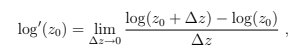We encounter an immediate obstacle: since the function log(z) is multiple-valued we have to make sure that the two log functions in the numerator tend to the same value in the limit, otherwise, the limit will not exist. In other words, we have to choose one of the infinitely many values for the log function in a consistent way. This way of restricting the values of a multiple-valued function to make it single-valued in some region (in the above example in some neighborhood of z0) is called choosing a branch of the function. For example, we define the principal branch Log(z) of the logarithmic function to be

`Log(z) = Log |z| + i Arg(z). `

The function Log(z) is single-valued, but at a price: it is no longer continuous in the whole complex plane since Arg(z) is not continuous in the whole complex plane. the principal branch Arg(z) of the argument function is discontinuous along the negative real axis. To see this, take z = -x + iε, where x>0 and ε tends to be 0 from two directions, that is, ε⇢0+ and ε⇢0-. When ε⇢0+, Arg(z) = π and when ε⇢0-, Arg(z) = -π. Hence, Arg(z) is discontinuous for all x<0. Hence, Log(z) is also discontinuous.

Let D denote all the points in the complex plane except for those which are real and non-positive; in other words, D is the complement of the non-positive real axis.  Log(z) is single-valued and continuous for all points in D. We will now check that it is analytic there as well. For this, we need to compute its derivative.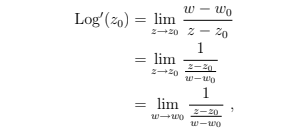where to reach the second line we used the fact that w = w0 implies z = z0 (single-valuedness of the exponential function), and to reach the third line we used the continuity of Log(z) in D to deduce that w → w0 as z → z0. Now using that z = ew we see that what we have here is the reciprocal of the derivative of the exponential function,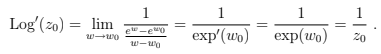Same result as in real calculus. This result holds good for all points in D, hence Log(z) is analytic in D.

Complex Power

With the logarithm function at our disposal, we are able to define the complex powers of complex numbers. Let α be a complex number. For all z ≠ 0, we define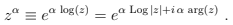The multiple-valuedness of the argument means that generically there will be an infinite number of values for zα. We can rewrite the above expression a little to make this manifest: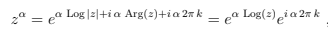where k is an integer.

My Personal Notes arrow_drop_up
Recommended Articles
Page :